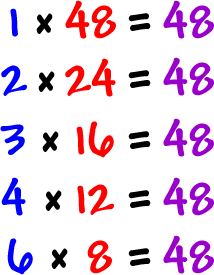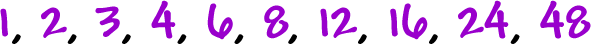Let's do one more:

Find the factors of 48.The factors of 48 areOK, one more:

Find the factors of 13.Hmm...  Looks like that's it!

The factors of 13 are 1 and 13.

TRY IT:

Find the factors of 10.

Find the factors of 32.

Find the factors of 19.

Find the factors of 100.